# Intermediate Bear tutorial

If you have not worked through the simple tutorial, where I show you how to run Bear on data that can be well modeled by linear regression, then I strongly recommend that you at least read through it first.

All the commands I use below are listed here.

## Nonlinear dependence

All of the bivariate datasets we looked at in the simple tutorial were generated with an underlying linear dependence of the label on the feature. Let’s immediately move to a dataset that is manifestly nonlinear, modifying our command for generating linear-5.csv by removing the linear weight term with --weight=0 and adding a quadratic term with --quadratic=-2: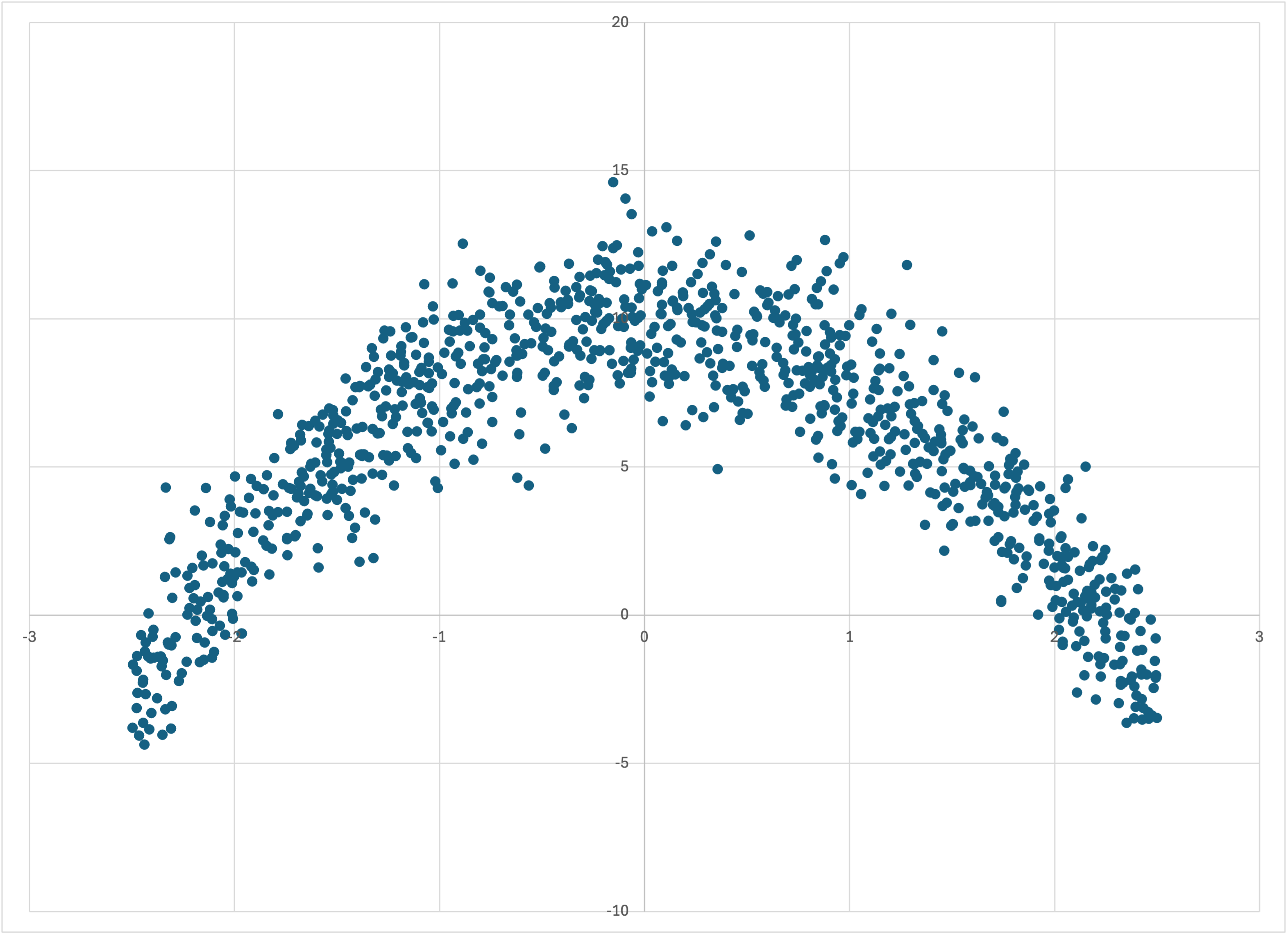If we just run memory_bear on this dataset in the same way that we did in the simple tutorial,

then we find that Bear finds a piecewise constant model that has five pieces that follow the quadratic relationship: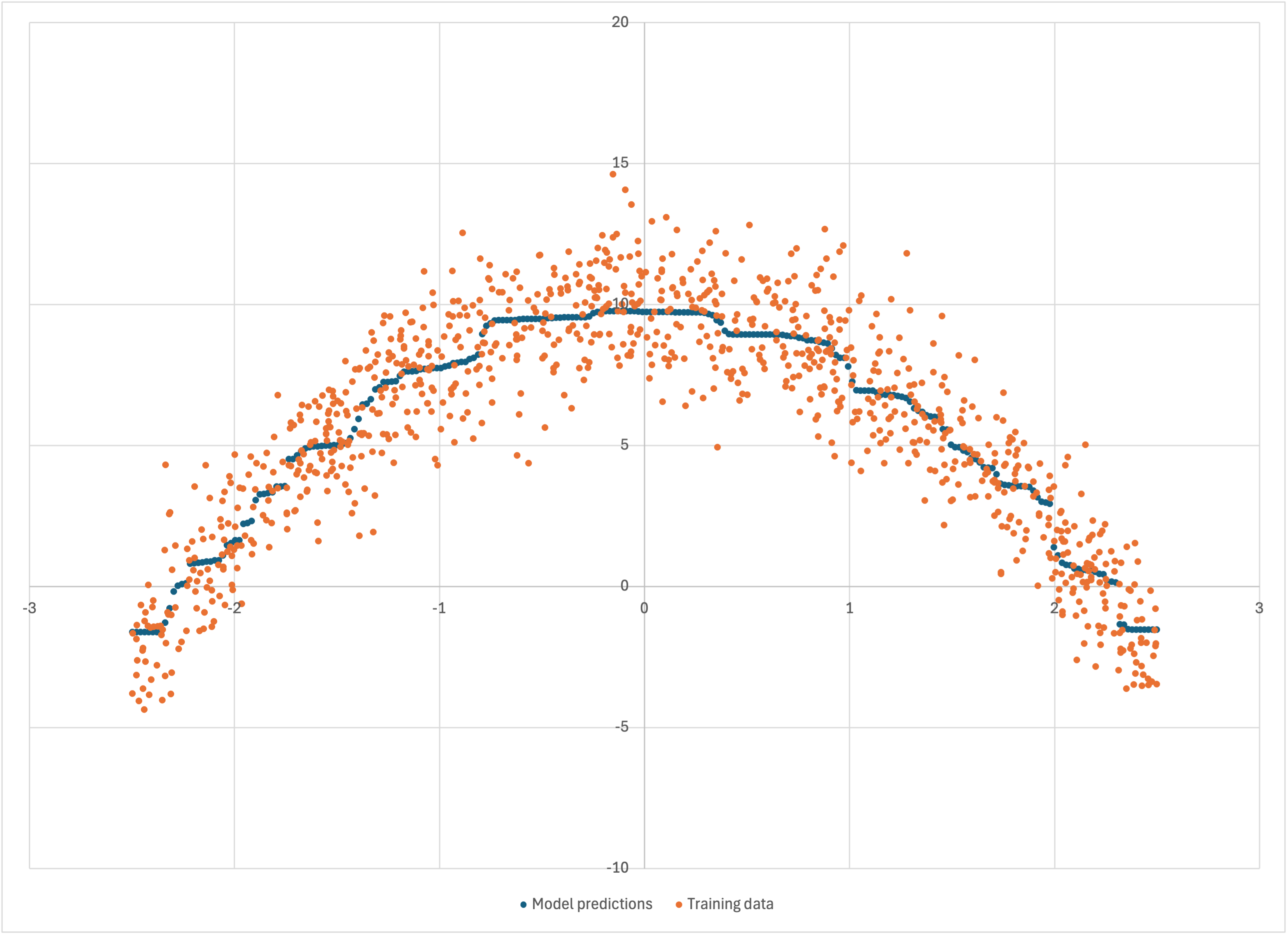So Bear models nonlinear data without us having to tell it what to do. The residuals are visually no worse or better than those of the linear model,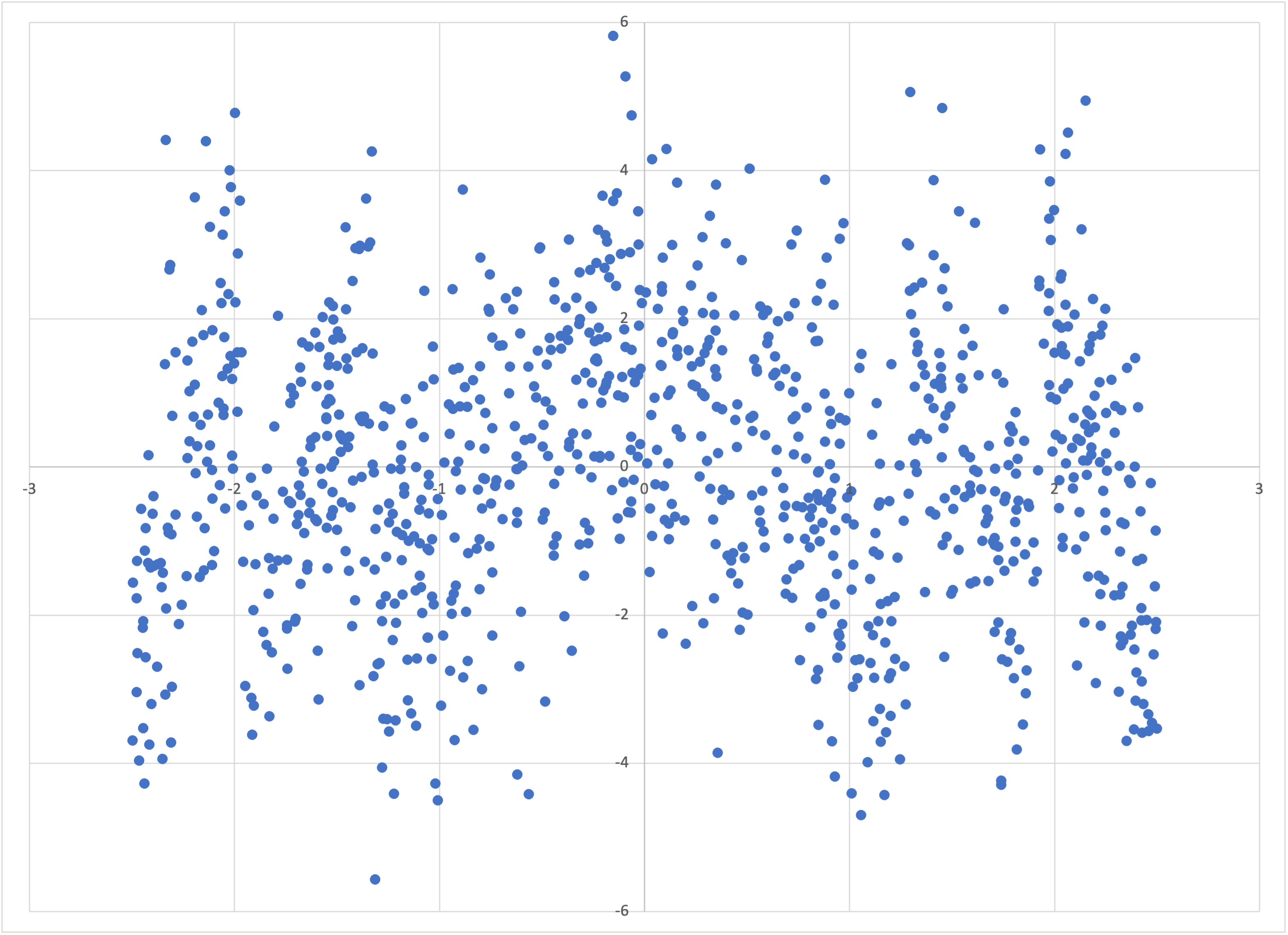and again it is not visually obvious how one could find statistical significance in any further partitioning of the feature variable.

## Binary classification

Let’s now turn to another type of problem that linear regression is not suited to: classification. For concreteness, consider the following hypothetical scenario: Imagine that we have data for three different types of house in a city in western Germany. Let’s label these three classes of house by the integers 4, 7, and 11. Let’s further imagine that we have surveyed a number of such houses, asking each if anyone in the house owns a particular brand of perfume. We put the results into the table perfume.csv: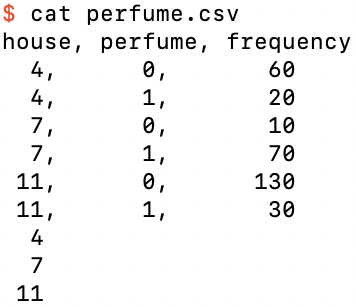The binary classification dataset perfume.csv

Note that this time I have included a header row of column names, as well as a frequency column that specifies the number of houses of house type house that gave the binary answer perfume to whether anyone in the house owned that brand. (I’ve made all the frequencies multiples of 10 just to make it easy to mentally do the math in the following.) I’ve also added three prediction rows, one for each of the house types 4, 7, and 11.

Let’s run Bear on this data. You can look up the help screen for the options to specify a header row and frequency column:

\$ memory_bear perfume.csv 1m -H -L perfume -f -C frequency -o perfume-predictions.tsv

yielding the predictions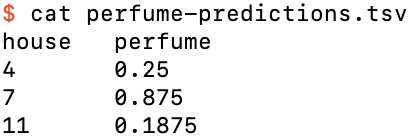Bear’s perfume-predictions.tsv

We see that the prediction for house type 4 is just 1/4, for type 7 is 7/8, and for type 11 is 3/16. Note that these are just the relative frequencies of the original data—namely, 20 out of 80, 70 out of 80, and 30 out of 160 respectively—or in other words, the empirical probabilities.

Bear predicts probabilities for classification data without us needing to do anything special.

## The loss function

You might, however, remember that Bear’s default loss function is MSE. Isn’t log loss usually used for classification problems?

It is easy enough for us to change to that loss function:

\$ memory_bear perfume.csv 1m -HLperfume -fCfrequency -operfume-log.tsv -nLOG

Although the “strength” that Bear reports for its model is slightly different (because its calculation is based on losses, as I will describe in the advanced tutorial), its predictions are the same as for MSE: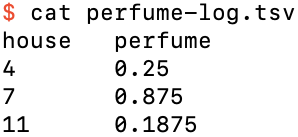Bear’s predictions using log loss

This is because for both MSE and log loss it is an elementary result that the predictions that minimize the loss function are the expectation values, here based on the empirical probabilities, and our data in this example has enough statistical significance that each category of house is modeled separately.

If we choose the balanced log loss function, on the other hand,

\$ memory_bear perfume.csv 1m -HLperfume -fCfrequency -operfume-balanced-log.tsv -nBALANCED_LOG

then the results are different: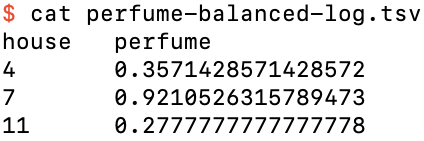Bear’s predictions using balanced log loss

This is because balanced log loss effectively ignores the actual fraction of labels that are 0 or 1 in the input data (here, 200 / 320 = 5/8 and 120 / 320 = 3/8), and “pretends” that they are actually equally likely. For this example, this effectively scales down the weight of all ‘0’ labels by a factor of (1/2) / (5/8) = 4/5, and scales up all ‘1’ labels by a factor of (1/2) / (3/8) = 4/3, so that, for example, for house type 4 the “effective frequencies” for ‘0’ and ‘1’ are 48 and 26⅔ respectively, so that the “effective mean” that it computes for house type 4 is 26⅔ / 74⅔ = 5/14 ≈ 0.3571, as shown in the table above.

Note that Bear performs this transformation automatically, without needing to throw away any of the actual input data in its calculations of statistical dependence and significance. (It actually calculates the same probabilities as with the other loss functions, and only transforms these into “estimates” that minimize the balanced log loss when you ask for its “predictions.”)

## The same data, but less of it

Let’s now look at the same dataset, but with all the frequencies divided by 10, in perfume-less.csv: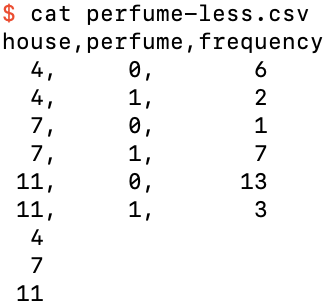The dataset perfume-less.csv

Running Bear on this data, using the default MSE loss,

\$ memory_bear perfume-less.csv 1m -HLperfume -fCfrequency -operfume-less-predictions.tsv

yields perfume-less-predictions.tsv: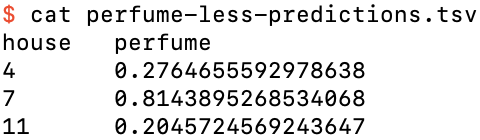The predictions for perfume-less.csv using MSE loss

In this case, Bear has clearly decided that it does not have enough data to conclude statistically significantly that the house types 4, 7, and 11 should be kept distinct, and that its predicted probability for each is just the overall relative frequency of 3/8. The same is true if we use log loss:

\$ memory_bear perfume-less.csv 1m -HLperfume -fCfrequency -operfume-less-log.tsv -nLOG

which yields perfume-less-log.tsv: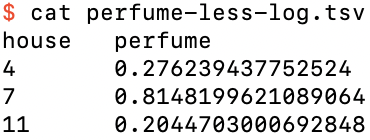The predictions for perfume-less.csv using log loss

And finally, balanced log loss,

\$ memory_bear perfume-less.csv 1m -HLperfume -fCfrequency -operfume-less-b-l.tsv -nBALANCED_LOG

which yields perfume-less-balanced-log.tsv,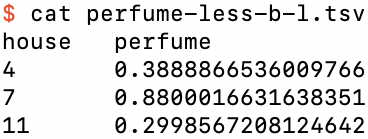The predictions for perfume-less.csv using balanced log loss

which, as expected, treats the binary variable perfume to be equally likely true or false.

## Missing data

Let’s go back to the full perfume.csv, and create perfume-missing.csv by adding two extra training rows for examples where we don’t know what the house type was, and an extra prediction row for that missing feature value: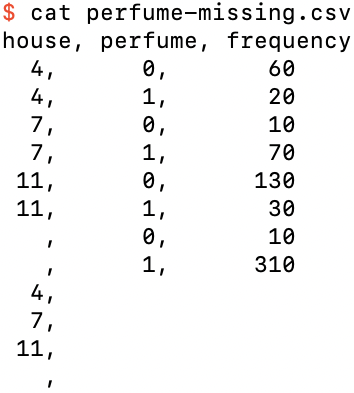The dataset perfume-missing.csv

Running this through Bear,

\$ memory_bear perfume-missing.csv 1m -HLperfume -fCfrequency -operfume-missing-out.tsv

it produces the expected results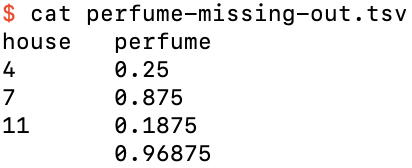The predictions perfume-missing-out.tsv

where the prediction if we are missing the house feature value is just 31/32.

## One-hot encoding

To this point we have encoded the three different categories of house type as the arbitrary numerical values 4, 7, and 11. A more standard way of representing this categorical feature is with one-hot encoding: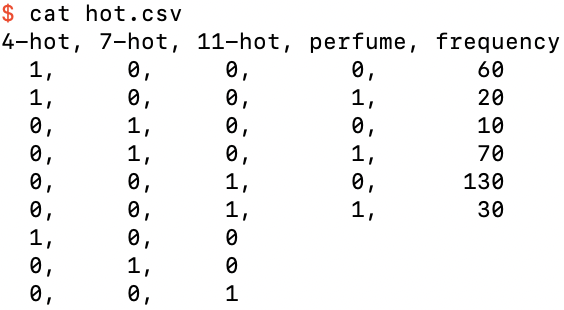The dataset hot.csv, a one-hot encoding of perfume.csv

where the categorical feature house has been replaced by the three binary one-hot features 4-hot, 7-hot, and 11-hot. We have now moved beyond bivariate data, because we have three features and one label, i.e., four variables in total. Regardless, we can still just ask Bear to model this data:

\$ memory_bear hot.csv -Hl3 -fc4 1m -ohot-predictions.tsv

We see that its predictions, in hot-predictions.tsv, are the same as for perfume-predictions.tsv: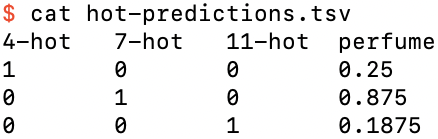The predictions file for hot.tsv

It looks like Bear has just done a four-dimensional generalization of what we saw it do in the simple tutorial. But if you inspect the logs for this run, it seems that Bear has actually done a lot more work just to come up with these predictions. In each of its two iterations it creates 3 “elementary” models, then creates between 100 and 300 (usually less than 150) “composite” models. After the second iteration it then “selects a final set of models to keep.” What is all this about?

As with the examples in the simple tutorial, Bear first constructs the empty model, which will just make the constant prediction 3/8 for any set of feature values. Next, Bear tries modeling the residuals of the empty model against each feature, alone, in bivariate models like the ones we worked through in the simple tutorial. In other words, it models the empty-model residuals against the first feature, here “4-hot,” and then it models them against the second feature, “7-hot,” and then against the third feature, “11-hot.” (It actually does these in parallel.) It calls these single-feature models “elementary models.”

For the bivariate examples of the simple tutorial, that’s all Bear had to do. But when there is more than one feature it has to do more.

First, it looks at all of its elementary models, and computes a “weight” for each of them, as we saw in the simple tutorial. (I’ll provide a mathematical description of this in the advanced tutorial.)

Next, it randomly selects one of these elementary models, where the random selection is weighted by these weights. This selected model is called the “base.” Bear then randomly selects again from all the elementary models, again weighted by the weight of each. This second selected model is called the “attachment.”

Bear then tries to “attach” the attachment to the base in two different ways:

1. with the attachment modeling the residuals of the base model, i.e., a bivariate model; and
2. with the base and attachment being “melted together” into a three-dimensional dataset containing the base’s feature, the attachment’s feature, and the residuals of the empty model, i.e., a trivariate model.

Within my codebase, the structural representation of each model is called a “part,” and the structural representation of the overall composite model created is called an “assembly”:

• The assembly for the empty model has no parts.
• The assembly for an elementary model has one part, which has one feature.
• In case (1) above the assembly has two parts, and each part has one feature.
• In case (2) above the assembly has one part, which has two features.

Bear has a number of heuristics aimed at preventing it from wasting time trying to create composite models that either don’t make sense or aren’t going to be useful. This is a subtle, because it is quite possible that a combination of features might be useful even if none of them individually are useful. It is also possible that a repeated part might be useful, but on the other hand we don’t want Bear to keep trying to repeat the same parts over and over ad infinitum.

Bear computes the “weight” of each of the composite models it creates, and adds them to its list of models. For the purposes of random selection, it only uses the excess of “weight” of a joined model over that of its base, i.e., its “value-add.”

And then it just repeats the process! Of course, it needs to sample without replacement, because there is no point in building a model that has already been built.

I described above how Bear tries to attach an elementary model to another elementary model. But what does Bear do when the base or the attachment has more than one part?

In this case, the first time that Bear selects a given attachment for a given base, it tries to glue or melt the first part of the attachment to the base. The second time that it selects that same attachment for that same base, it tries to join the second part of the attachment (if it has at least two parts) to the base. When all attachment parts have been tried for that combination of base and attachment, that given combination of base and attachment is “vetoed,” so that it is not tried again.

My logic here is that models that have been found to be useful are probably likely to contain parts that lead to better models when attached to a good base model. However, my algorithm doesn’t just use a greedy selection, because, as I noted above, features or parts may be relatively weak or even useless by themselves, but become useful in combination with others. On the other hand, the random selection is weighted towards those models that have shown themselves to be better (over their bases) than others, because for more than a small number of features the combinatorial explosion means that it is impossible to try every possible combination of features.

Bear continues this process until there are no more attachments possible between the models that it has created that satisfy its usefulness heuristics, or until it runs out of time (which you specify when you run Bear).

Bear’s final task is to figure out which of those constructed models it should use for predictions. It first sorts them in decreasing order of weight (and, where equal, increasing “complexity”). It then goes through this list, collecting those models that it finds to be useful. A model is deemed to be useful when its predictions, added to those of all models collected so far, with each weighted by the given model’s weight, results in a lower overall loss. (I will make this more specific in the advanced tutorial.)

For our example above, it seems like Bear only chose one model for our dataset, because its predictions are precisely what you would get from the relative frequencies of the training data. Does this mean that its model included all three of our one-hot features? We can check this either by saving Bear’s model as we did in the simple tutorial, or by using the --details-filename optional argument (which, doesn’t, however, give us the option for verbose mode):

\$ memory_bear hot.csv -Hl3 -fc4 1m -ohot-predictions.tsv -Dhot-details.csv

which yields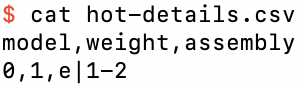The details file for hot-details.tsv

The notation e|0-1 signifies that Bear’s single model used features 0 and 1 to model the residuals of the empty model. It didn’t use feature 2 at all. How can this be?

Well, one-hot encoding contains a redundancy: one of the features has to be hot. Thus, any of the models e|0-1, e|0-2, and e|1-2 that uses two of the three features is just as good as the model e|0-1-2 that uses all three. By its simplicity criterion, Bear chooses one of these simpler models, rather than the model that uses all three features. Indeed, if you run Bear multiple times, you will see that it chooses which two features to use in its final model randomly.

## One-hot with less data

If you create the one-hot encoded version of perfume-less.csv, namely, hot-less.csv: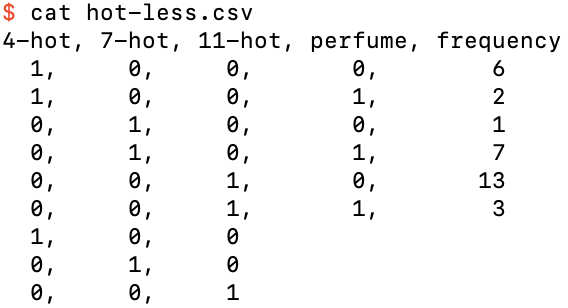The dataset hot-less.csv, a one-hot encoding of perfume-less.csv

then if you run Bear on it,

\$ memory_bear hot-less.csv -Hl3 -fc4 1m -ohot-less-predictions.tsv -Dhot-less-details.csv

we find that its predictions in hot-less-predictions.tsv are the same as for perfume-less-predictions.tsv, namely, just the overall relative frequency: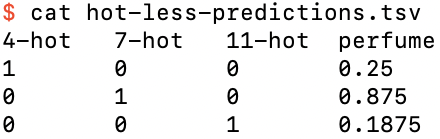The predictions for hot-less.csv

which is reflected in Bear’s model being just the empty model: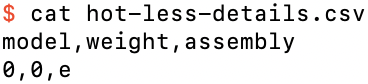The model for hot-less.csv

## Trivariate nonlinear data

We have seen above that Bear can directly model continuous but nonlinear bivariate data, which we can easily visualize on a scatterplot, and binary classifcation data with either a categorical feature or multiple one-hot features, which we can view easily in tabular form. What about continuous nonlinear data with more than one feature?

As a simple but nontrivial example, let’s assume that we have trivariate data with one label z that depends on two features x and y. For simplicity, imagine that the true z is equal to 2.5 within an annulus (ring) of outer diameter 5 and inner diameter 3 in x–y space, and zero otherwise:The true value of z is 2.5 in the blue annulus and zero in the white areas

We can make our visualization of z(x, y) concrete by cutting off a 2.5 mm length of brass tube that has a 5 mm outer diameter and 3 mm inner diameter: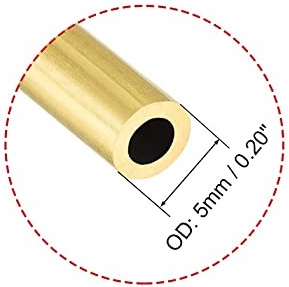The brass tube that helps us visualize z(x, y)

We place this piece of brass tube on the ground somewhere:The piece of brass tube, sitting on the ground

The value of z at any (x, y) position is the height of the top of the piece of tube, 2.5 mm, or the ground level z = 0 mm for those (x, y) positions that have no brass above them.

The piece of brass tube actually looks similar to the Hirshhorn Museum:The Hirshhorn Museum

except that it doesn’t have the Hirshhorn’s “stilts” at its base. You can use either physical object to help you visualize z(x, y).

To make our mathematical representation of this piece of brass tube visualizable without special software, we will use x and y coordinates restricted to a square grid. Bear doesn’t require this; it just means that we can use a program like Excel (or any other program you choose to use) to create a surface map for us. On the other hand, we can still add some gaussian noise to the height z to represent real-world measurement noise.

If you have built Bear, then you have a program that will generate this data file for you:

\$ intermediate_bear_tutorial_data tube.csv 255 tube-x.csv 50 -r 19680707

The first argument, tube.csv, is the name of the file that will be created with this trivariate data in it. The second argument, 255, specifies how many samples will be on each side of the regular x–y grid; I have chosen 255 because that’s the maximum side length that Excel can handle for a surface chart. Thus the file tube.csv contains 255 x 255 = 65,025 rows of trivariate training data representing the height of the piece of tube or the ground, as the case may be. It then contains another 65,025 rows with the same (x, y) values as testing data: for simplicity, we are only asking Bear for its predictions for the same x–y grid (although of course in practice we could ask for predictions at any values of x and y).

In addition to this, the program creates a dataset representing the x–z projection of the full (x, y, z) dataset, that I will use below. (By rotational symmetry, the y–z projection is fundamentally the same.) The third argument, tube-x.csv, specifies the filename of this projection, and the fourth argument, 50, the number of samples per side for it; it will be useful for this to be both smaller than and greater than what we use for the full trivariate dataset. Because we don’t have the restrictions of Excel surface charts for this bivariate x–z projection data, the x values are randomly dithered, which will also help with the visualizations below.

Let us start with the bivariate x–z projection dataset tube-x.csv. Opening it in Excel,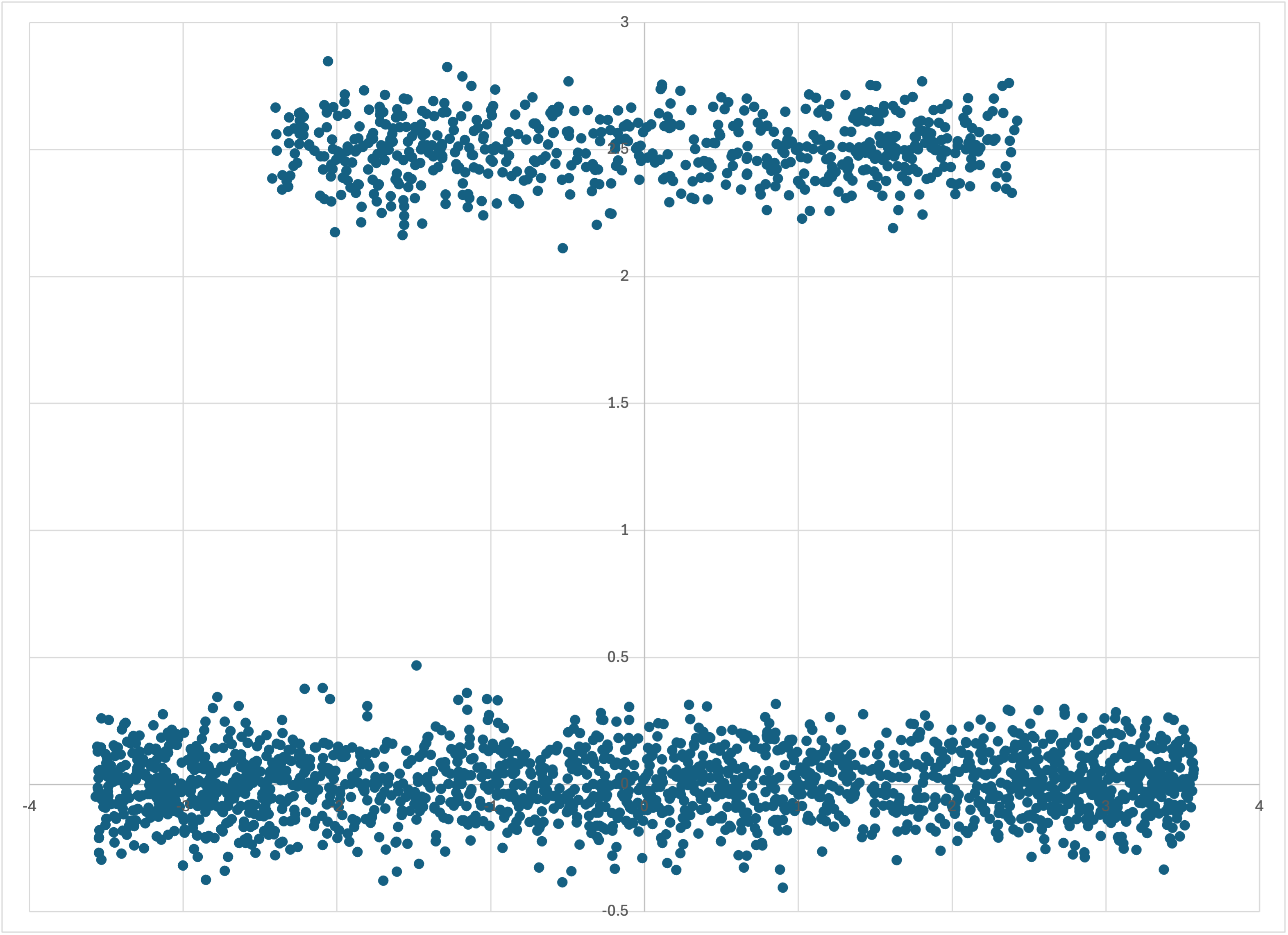The data in tube-x.csv

we can see immediately why this dataset would be a challenge for machine learning. Its symmetry in x means that linear regression would have been useless from the outset. But it’s clearly also bifurcated according to some “hidden” variable—which we know is just the depth, y. If we run Bear on this dataset in debug mode,

\$ memory_bear tube-x.csv -dl1 1m -otube-x-predictions.csv

then we see that its predictions, in tube-x-predictions.csv, struggle to “see” more than the fact that z values seem to be higher, on average, in the middle of the x range: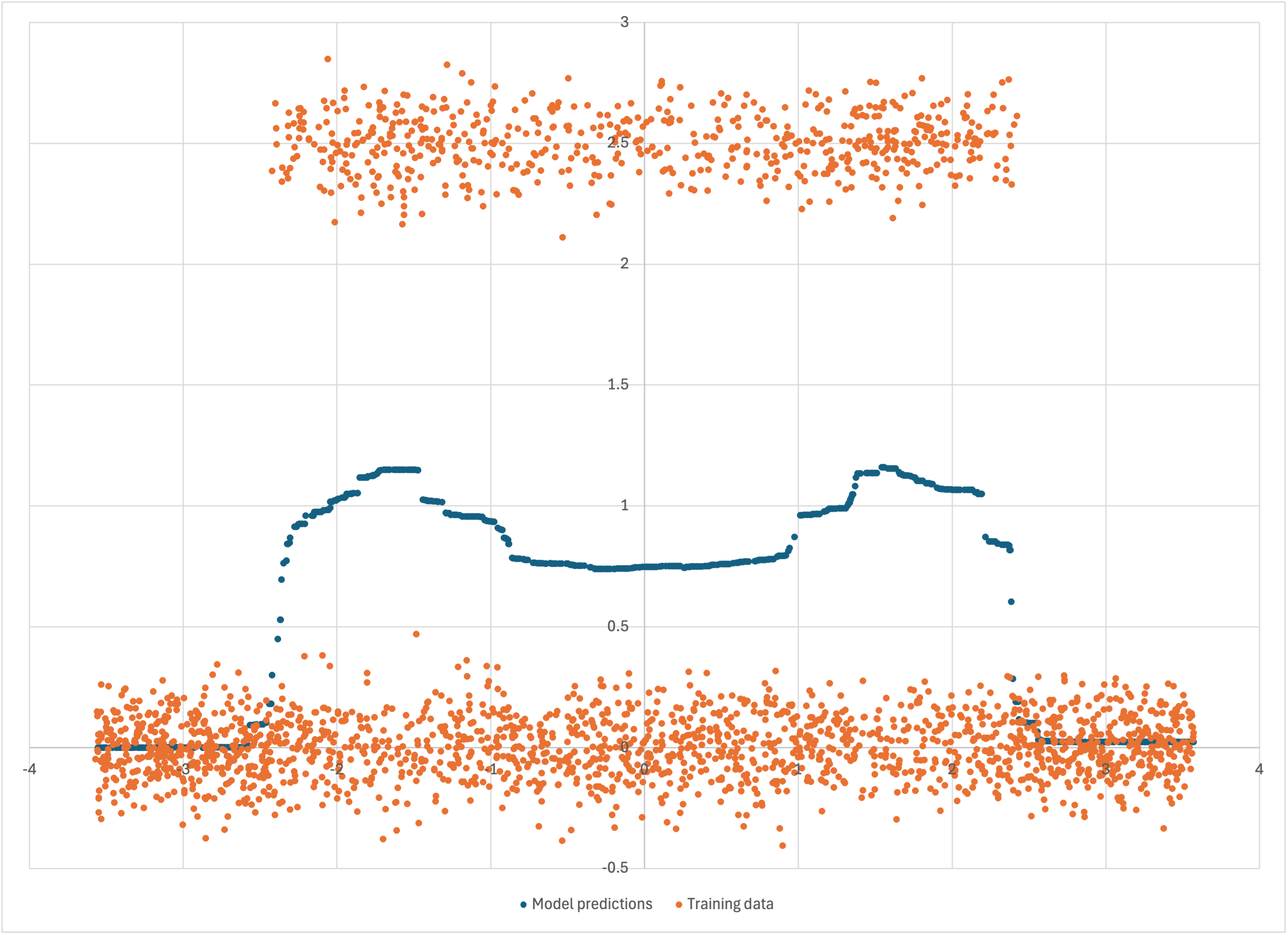Bear’s predictions for tube-x.csv, with the training data

Of course, we only used a 50 x 50 grid for this projection, so that we can actually see the data. Let’s create an x-projection dataset on a 1000 x 1000 grid instead, i.e., with a million data points, not just 2,500:

\$ intermediate_bear_tutorial_data /dev/null 2 tube-x-1000.csv.gz 1000 -r19680707

and run Bear on the file tube-x-1000.csv.gz, not in debug mode (since we don’t want to try to graph those million data points):

\$ memory_bear tube-x-1000.csv.gz -l1 1m -otube-x-1000-predictions.csv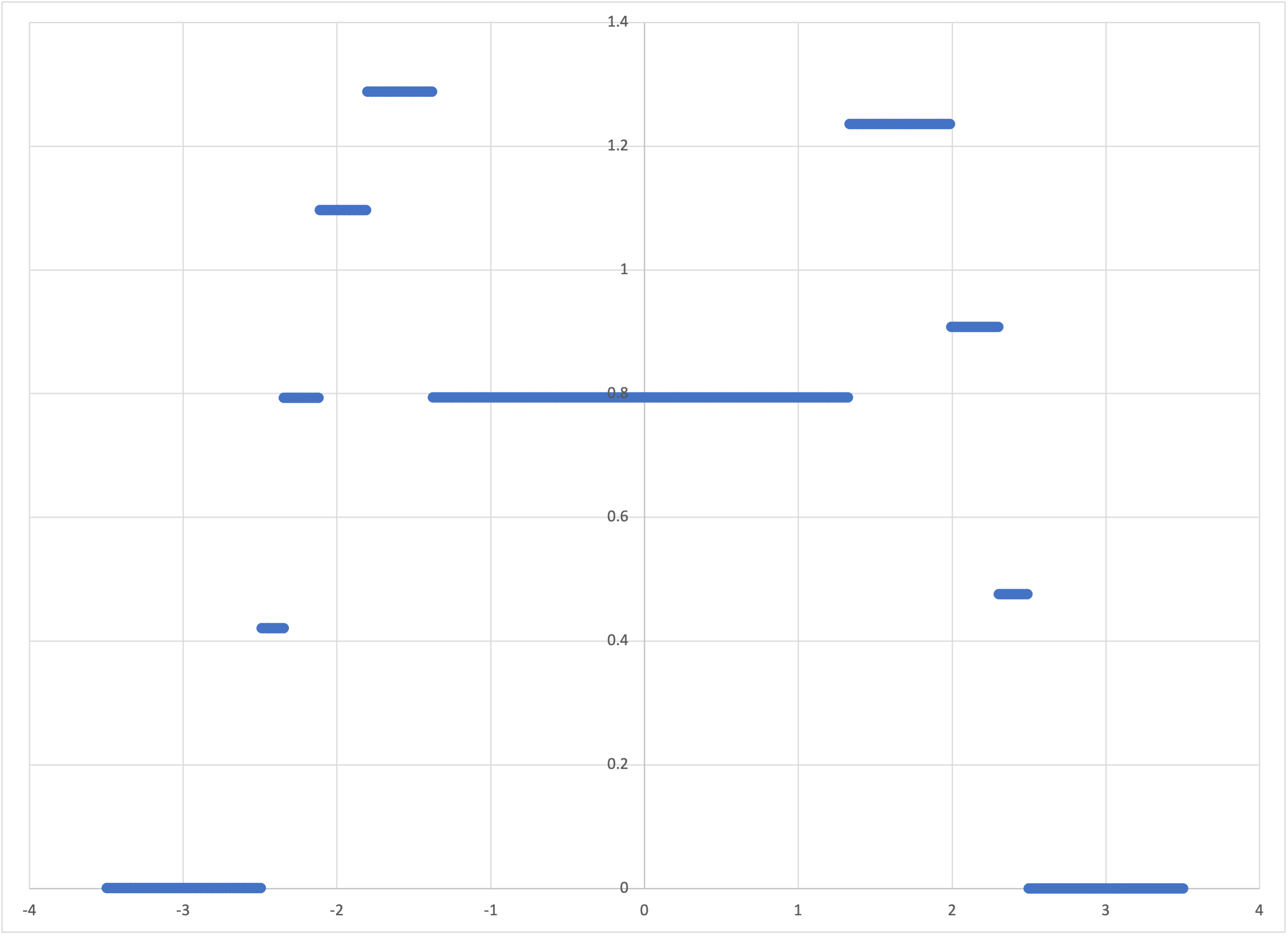Bear’s predictions for tube-x-1000.csv

The vast increase in data has allowed it to pick up that the “edges” of this “brass tire” (or “wagon wheel”) are denser, when viewed side-on, than the middle (and that this density increases as you move from the “outside wall” of the tire to the “inside wall”). This model represents with higher resolution the mean value of z as a function of x.

By circular symmetry, we know that the y–z elementary model is going to look the same.

Let’s now turn to the full trivariate dataset, tube.csv. I supply another program that reformats this data into a form that we can directly graph using Excel:

\$ intermediate_bear_tutorial_reformat tube.csv 255 tube-excel.csv

This converts the 255 x 255 = 65,025 training rows into a file tube-excel.csv with 255 rows and 255 columns, where each cell contains a z value. This file can be visualized as a surface chart in Excel (or you can use whichever graphing program you like):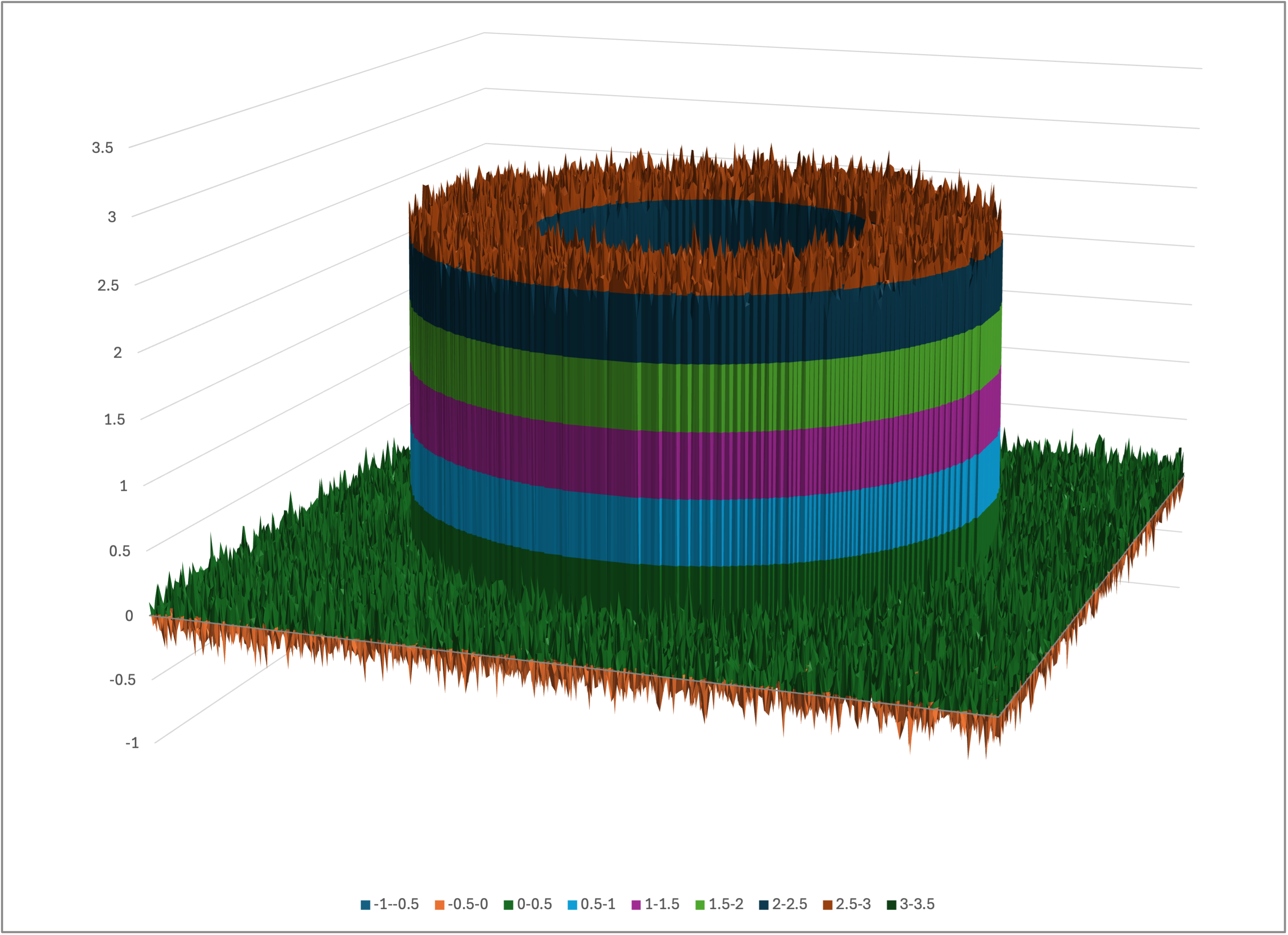Excel’s surface chart of tube-excel.csv

Rotating the view, you can look down into the tube: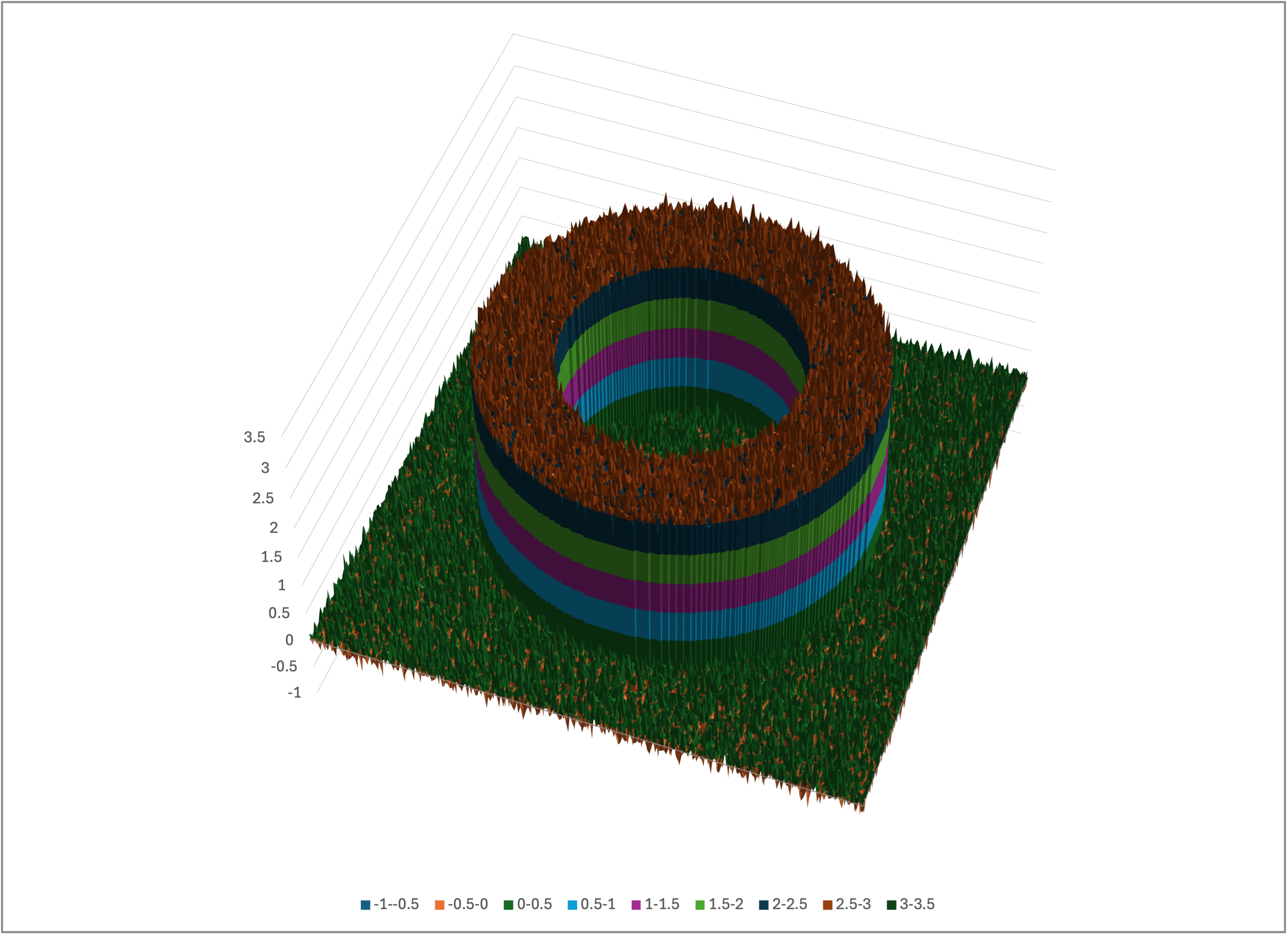A rotated view of tube-excel.csv

Let’s run Bear on this dataset:

\$ memory_bear tube.csv -l2 2m -otube-predictions.csv -stube

We can reformat the predictions file tube-predictions.csv using the same program as above:

\$ intermediate_bear_tutorial_reformat tube-predictions.csv 255 tube-predictions-excel.csv

Opening tube-predictions-excel.csv in Excel (or whatever you are using) and creating a surface chart of it, you should see something like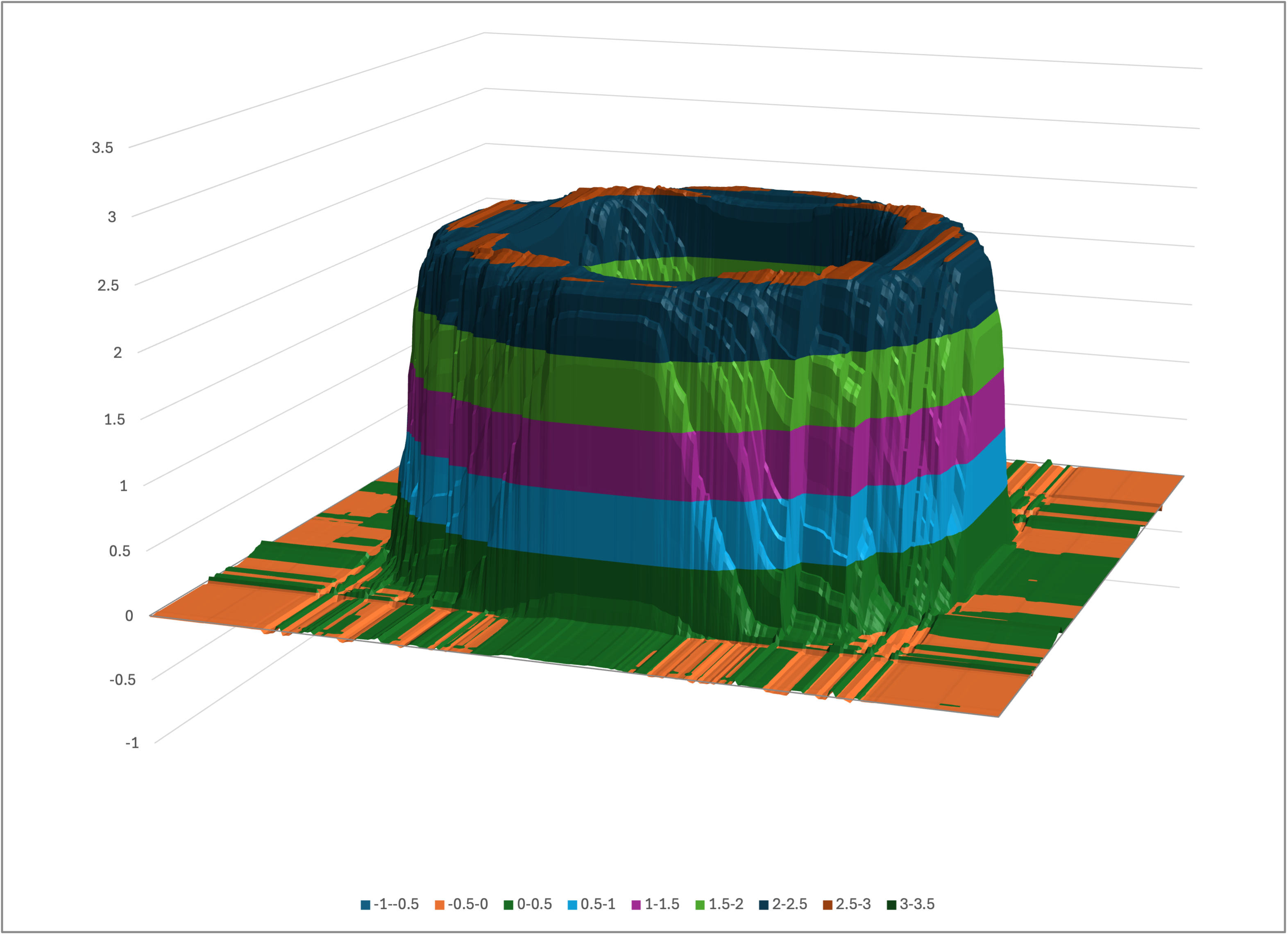Excel’s surface chart of tube-predictions-excel.csv

Again rotating, we can look down into the tube: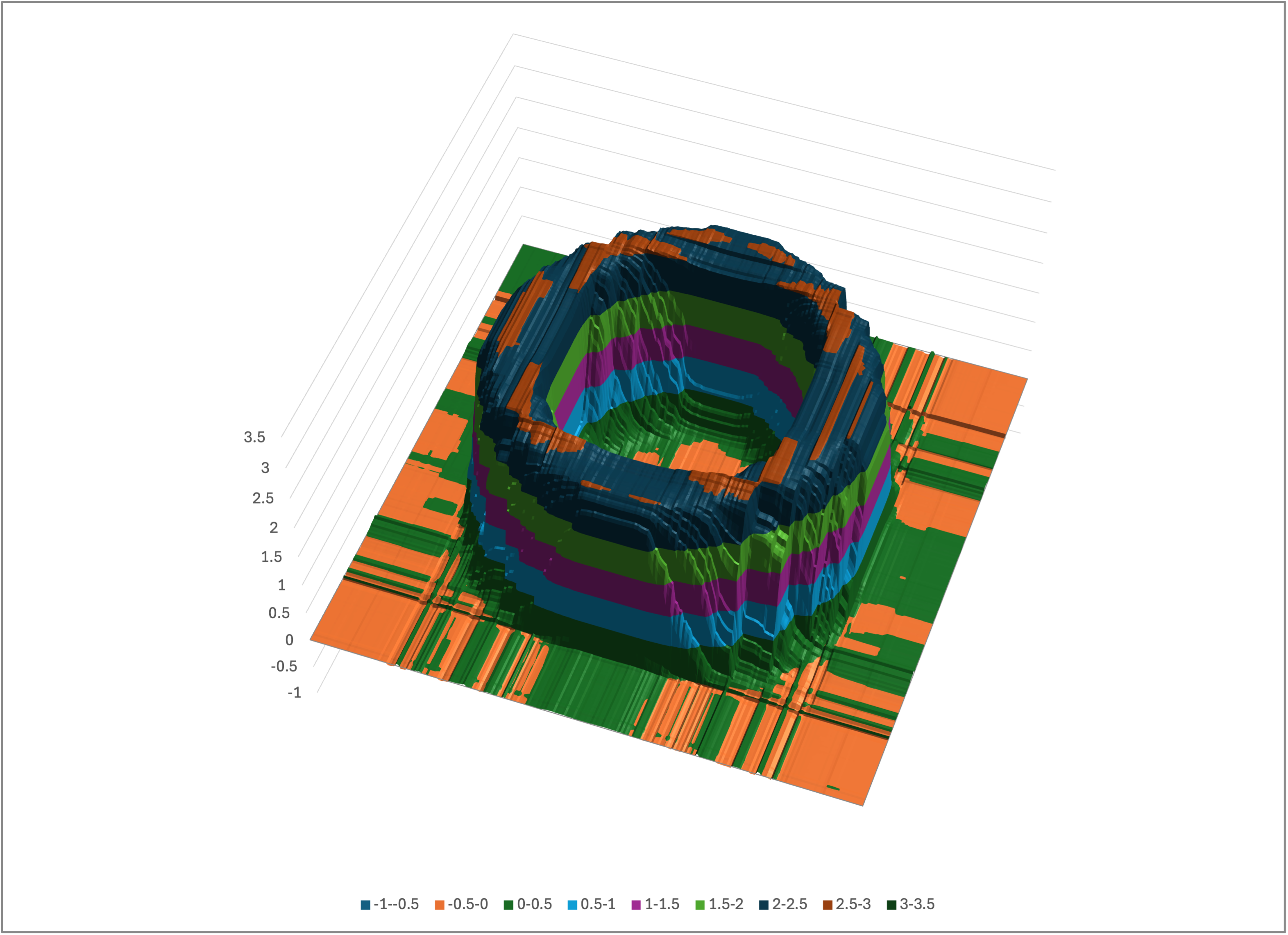A rotated view of tube-predictions-excel.csv

Even though it looks like Lego or Minecraft, Bear has arguably done a pretty good job!

It’s not magic, though. Bear had over 65,000 data points available to it for this model, which gave it enough statistical significance to model the full three-dimensional cube, as you can see if you look at the model file tube.bear.gz: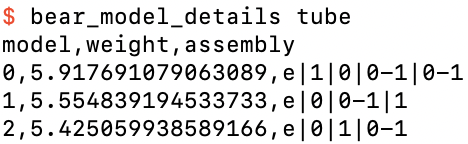The details of the model file tube.bear.gz

The models you see will generally vary slightly from the ones shown here, due to random tie-breaking choices made by Bear, but in general you will see that both features are used, singly and together, and sometimes in repeated parts.

If you look at tube.bear.gz you will see that it is only 5 or 6 KB in size. Compression only explains a small part of this efficiency: even uncompressed, it is not much larger. Bear’s model efficiency comes from having piecewise constant models, together with the internal use of the paw format which limits the cardinality of real-valued input fields.

## A noiseless tube

In the above we added noise to the representation of the tube, to reflect the real world. Let’s instead create a dataset with no noise:

\$ intermediate_bear_tutorial_data tube-nn.csv 255 /dev/null 50 -r 19680707 -e0
\$ intermediate_bear_tutorial_reformat tube-nn.csv 255 tube-nn-excel.csv

As expected, the tube is now noiseless: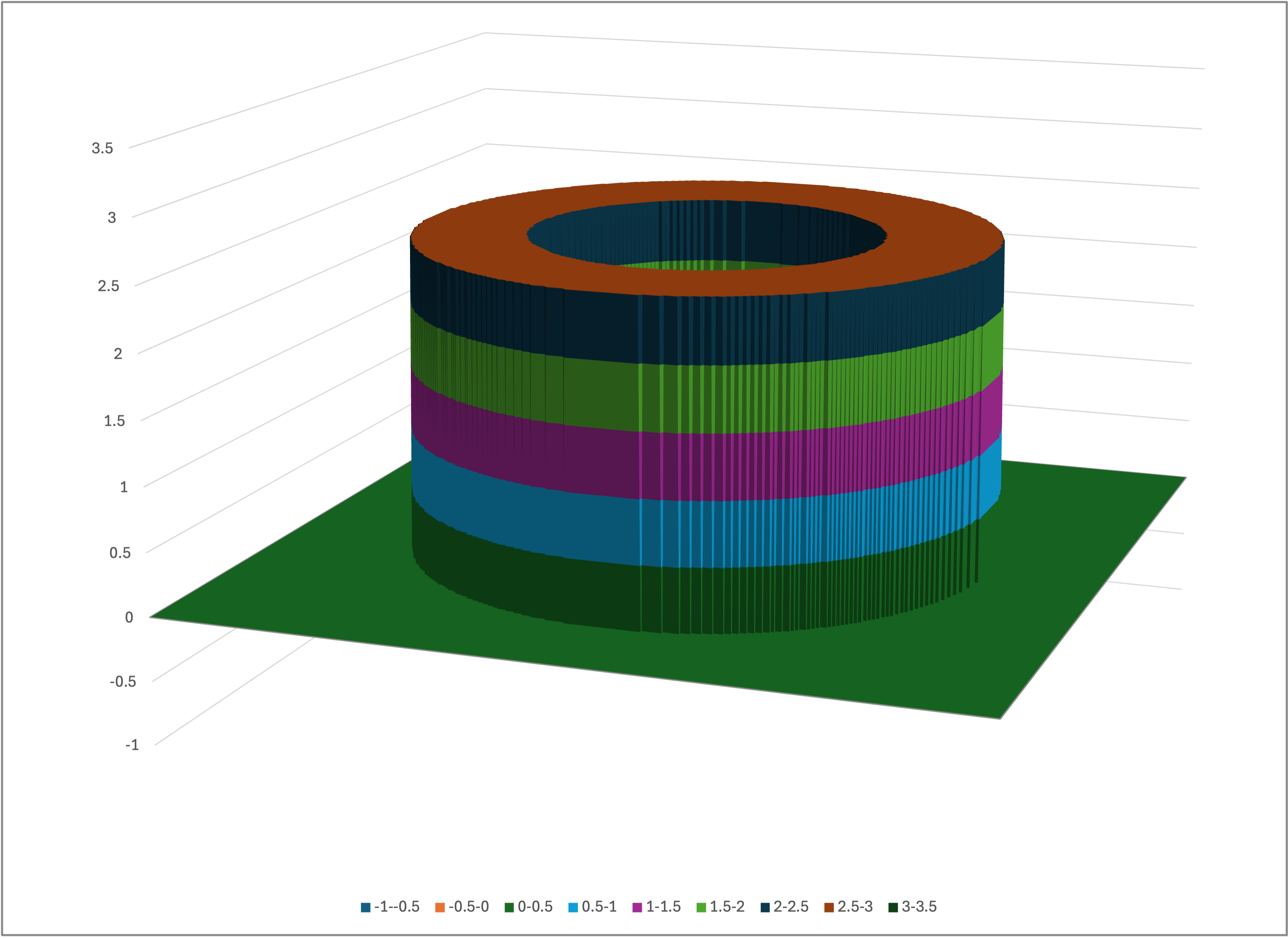Excel’s surface chart of tube-nn-excel.csv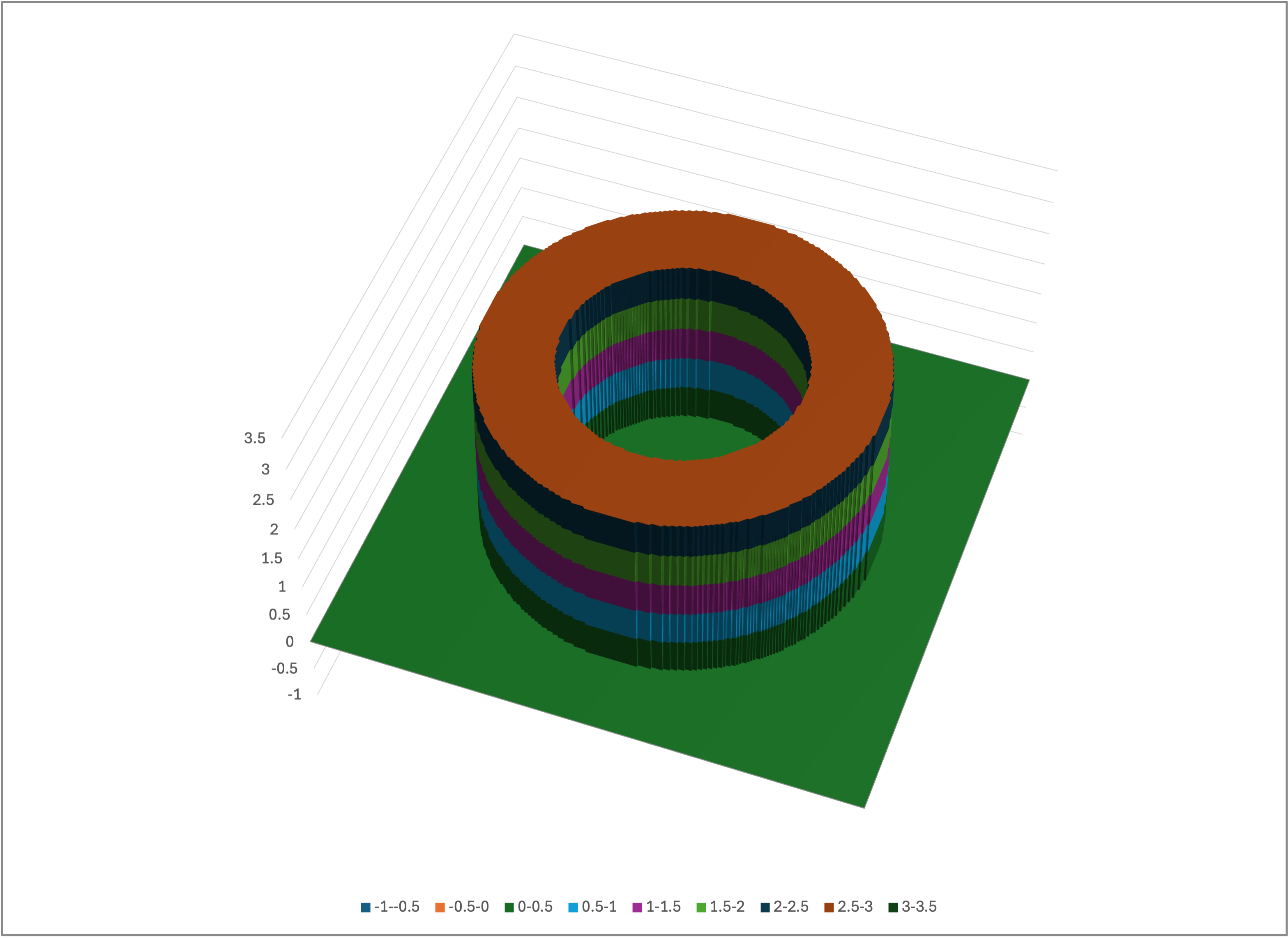A rotated view of tube-nn-excel.csv

If we run Bear on this noiseless data,

\$ memory_bear tube-nn.csv -l2 2m -otube-nn-predictions.csv -stube-nn
\$ intermediate_bear_tutorial_reformat tube-nn-predictions.csv 255 tube-nn-predictions-excel.csv

and look at the results,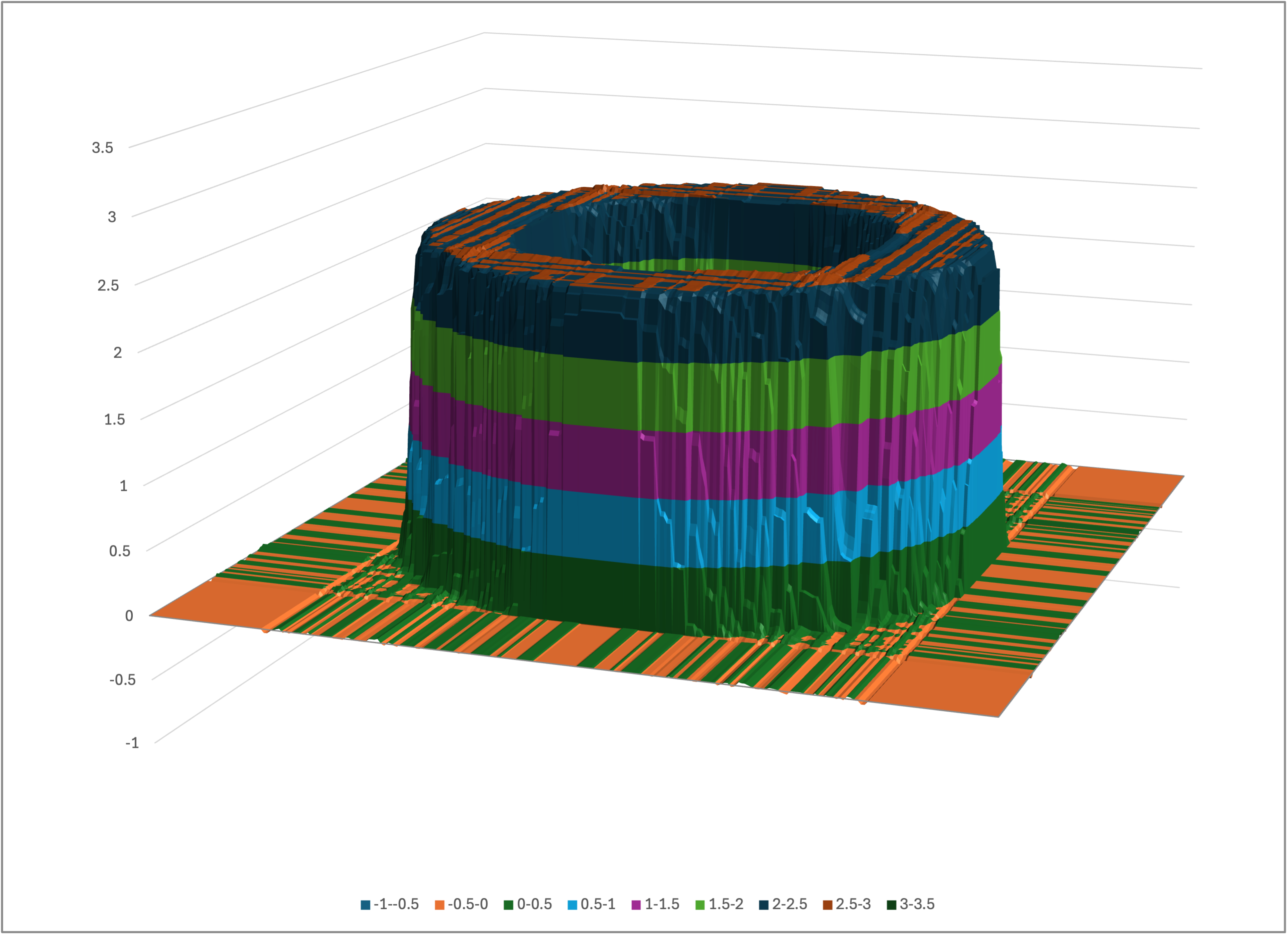Excel’s surface chart of tube-nn-predictions-excel.csv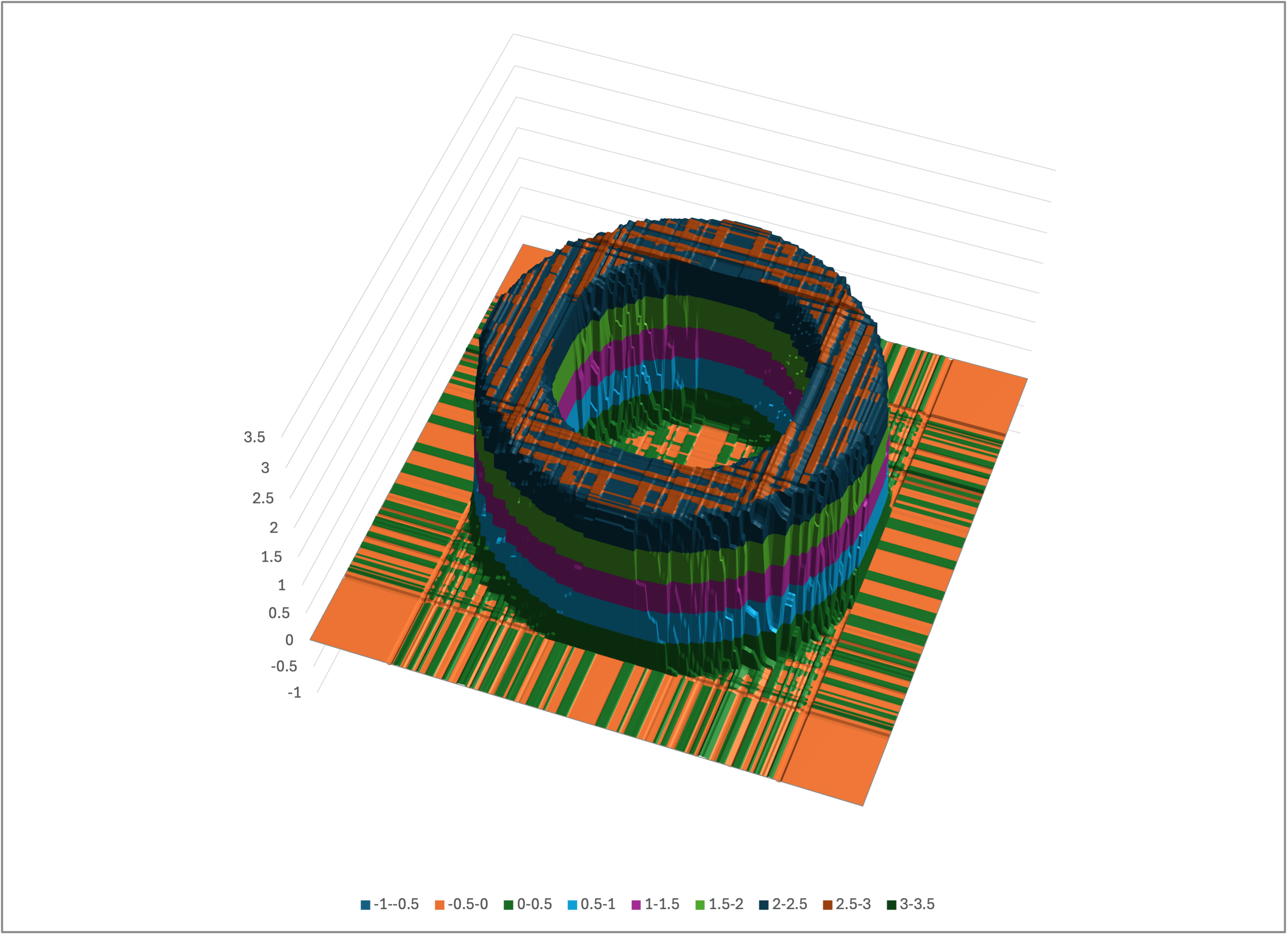A rotated view of tube-nn-predictions-excel.csv

we see that Bear’s modeling is qualitatively the same as it was for the noisy data. This again highlights that Bear does not overfit to noise in the data. Its estimates will always, of course, be somewhat “wobbly” due to that noise, but it shouldn’t completely “hallucinate” gross structure that isn’t really there.

## Less data for the tube

Let’s now return to my comment above that we gave Bear a relatively generous 65,000-odd data points to model this tube structure. If we add the noise back in, and decrease the number of data points to, say, 70 x 70 = 4900 points, Bear still does a pretty reasonable job: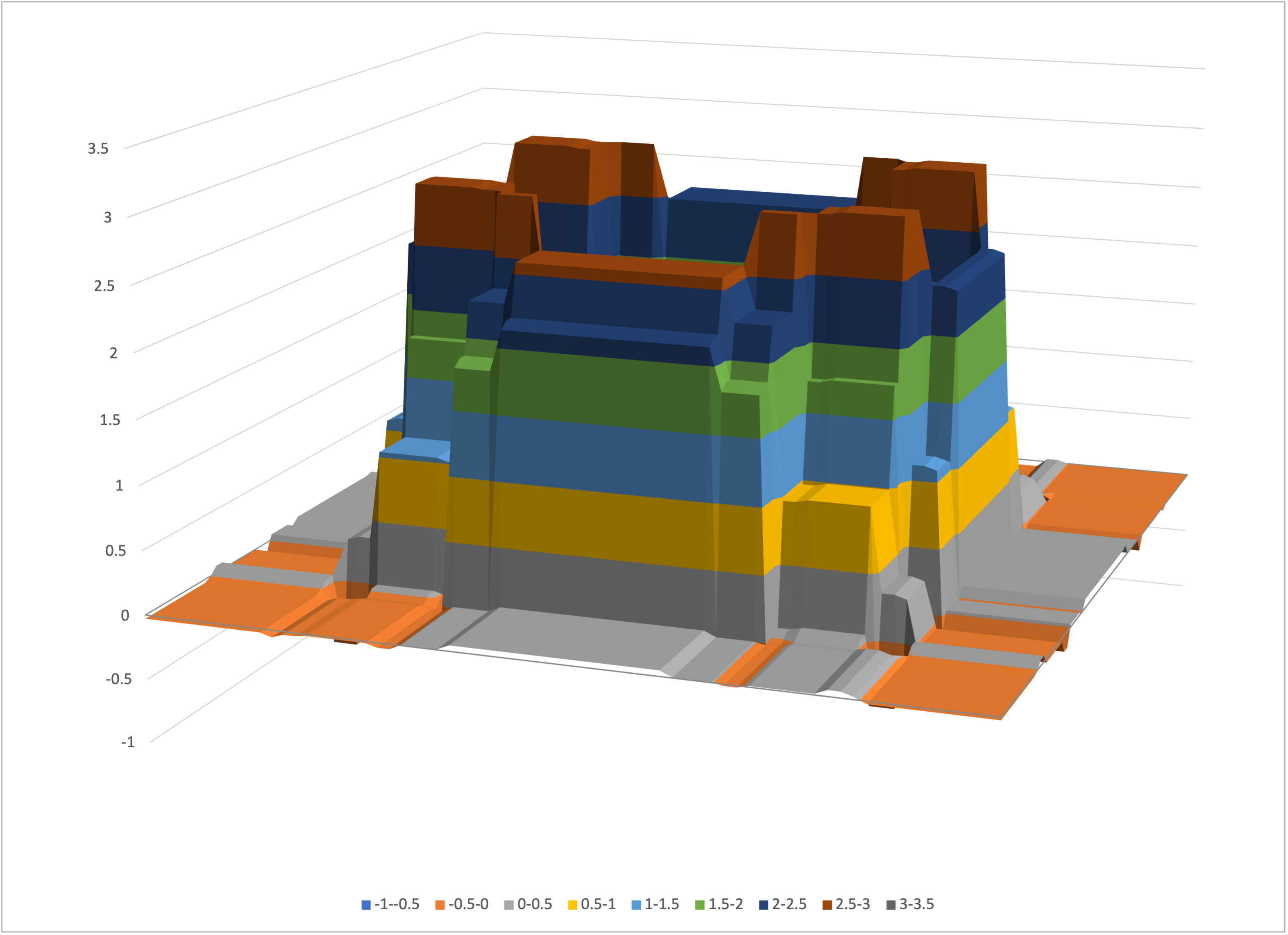Bear’s model of the tube with 70 x 70 data points

Even if we decrease further to 30 x 30 = 900 data points, it still manages to hang on: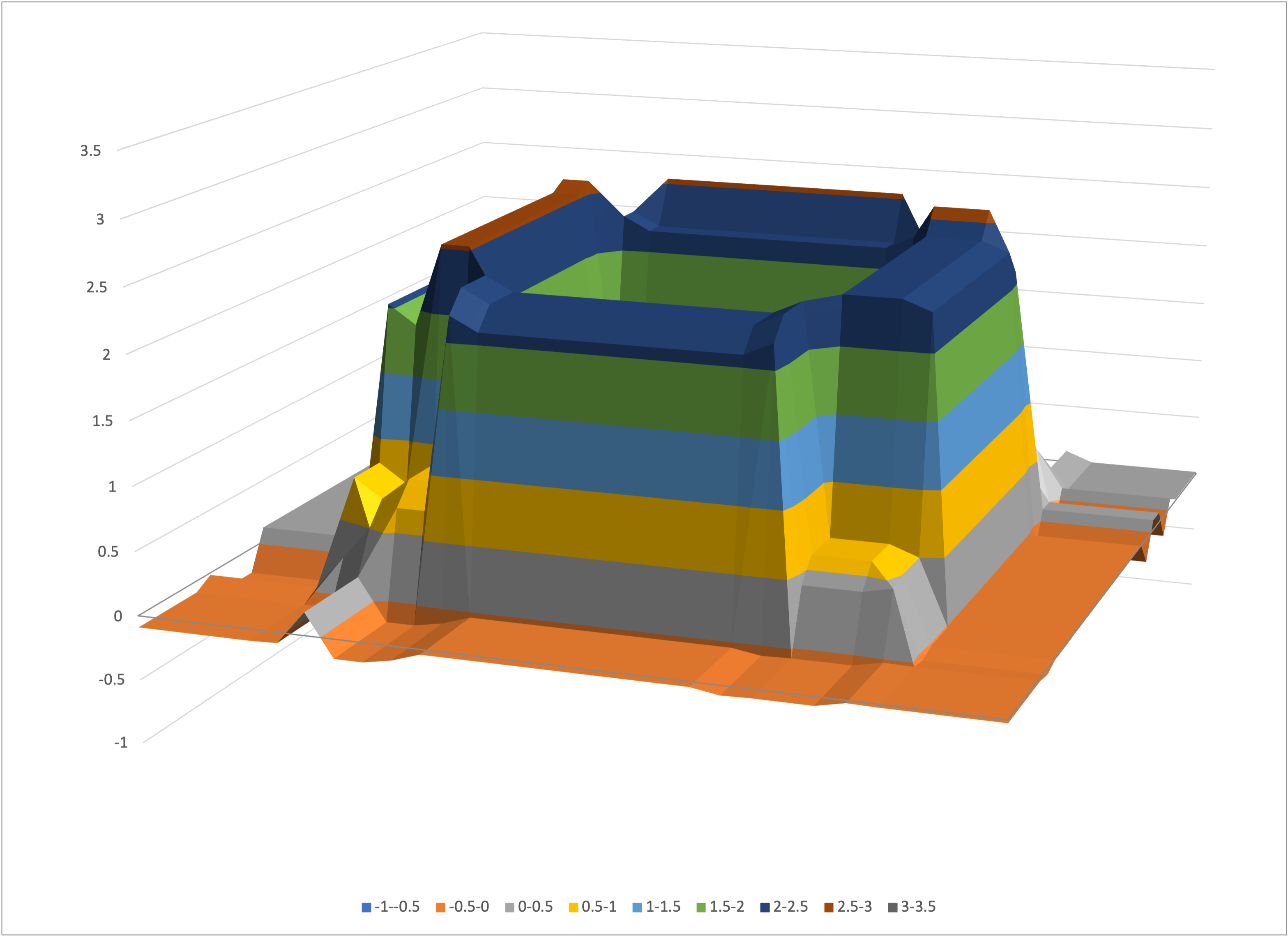Bear’s model of the tube with 30 x 30 points

At 20 x 20 = 400 data points, we have lost the “hole,” and the remaining object is “averaged out”: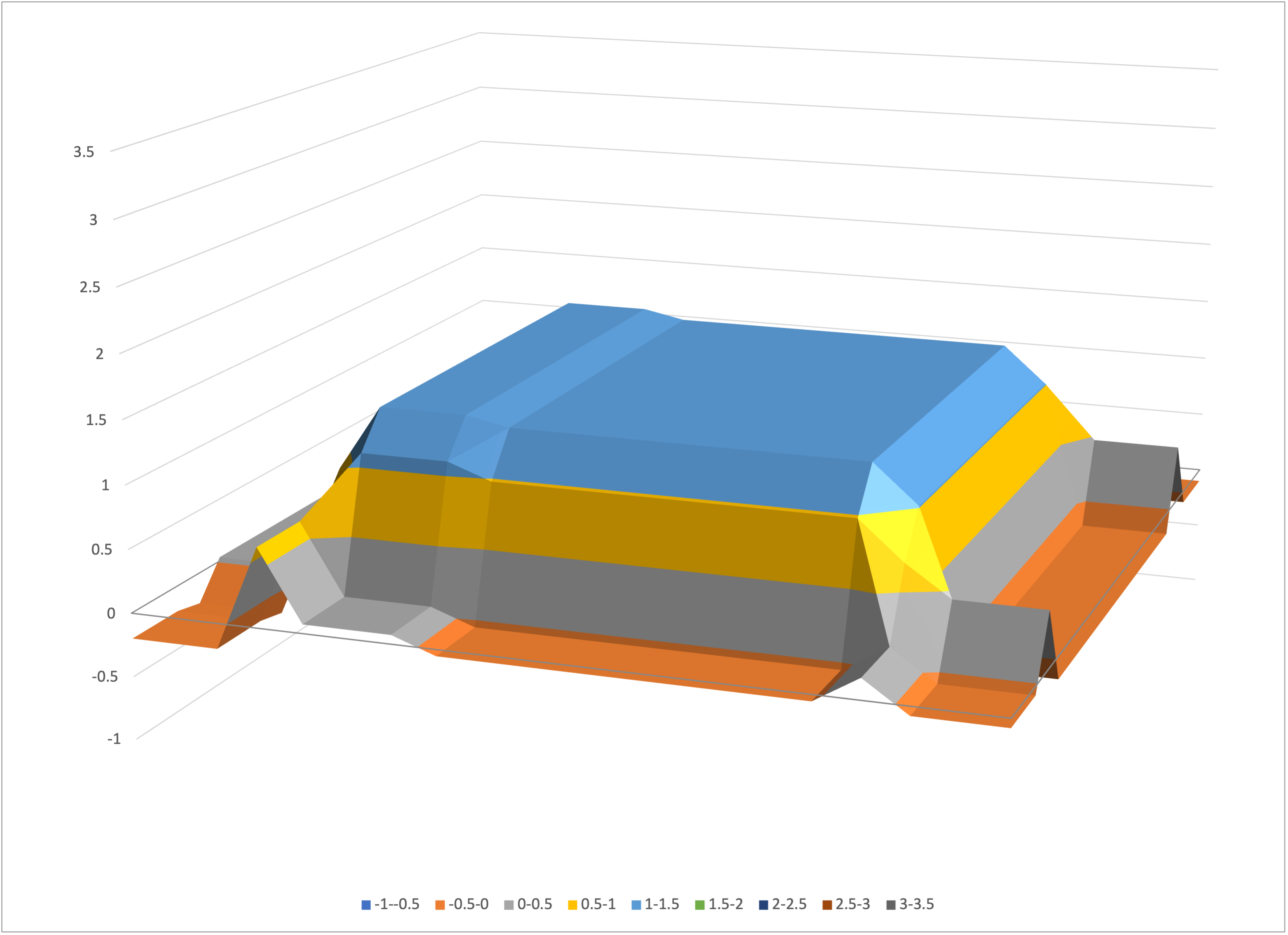Bear’s model of the tube with 20 x 20 points

Still, Bear has done the best that it can with the data available, and has not overfit to the noise.